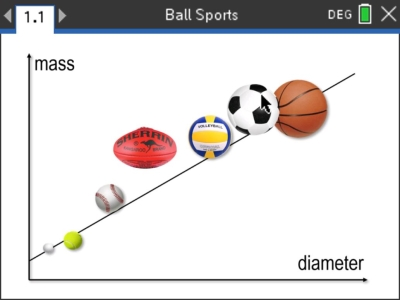# Activities

•• ##### AuthorMiddle

40 Minutes

• ##### Device
•TI-Nspire™ CX
•TI-Nspire™ CX CAS
• ##### Software

TI-Nspire™
TI-Nspire™ CAS

5.0

## ACMMG271 Ball Sports#### Activity Overview

In this activity students collect data for a range of ball sizes and masses. The data is then analysed and an appropriate line of best fit is applied. Students then consider balls that don't quite fit the plot, including an AFL football. In considering an AFL football students use alternative means to create a comparison with the spherical balls. Calculations include arithmetic mean (radius), geometric mean (radius) and volume. Students are ultimately provided with a problem involving the design of a ball using a specific material and thickness leading to calculations of density.

#### Objectives

Students use data to find a relationship between diameter and mass for commonly used sports balls. This information is then used in the design of a new ball given the density and thickness of the material being used. Students perform calculations of volume, density, mass and radius. Students also need to develop ways to analyse balls that are not spherical, such as an Australian Rules Football.

#### Vocabulary

• Volume
• Density
• Diameter
• Sphere
• Ellipsoid
• Least Squares Regression
• Arithmetic mean
• Geometric mean